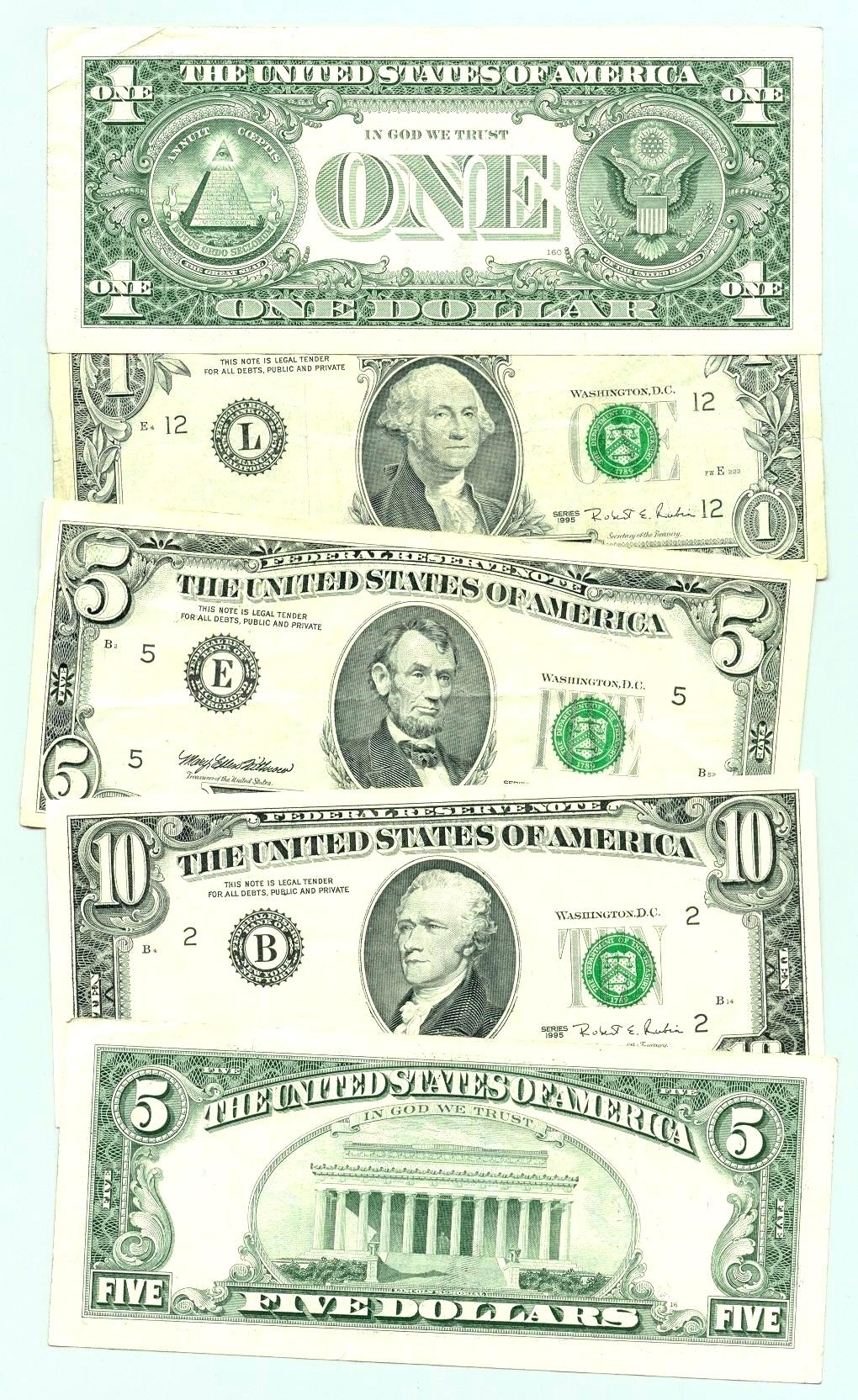Subtraction
Place Value
Time
Money
100

10+11 =

21

100

7-3=

4

100

Which number is in the ones place, 524

4

100What time is it?

3:00

100

Name this coin...Quarter

200

9+9 =

18

200

11-2=

9

200

which number is in the hundreds place, 2367

3

200What time is it?

10:10

200

Name this coin...dime

300

25+25 =

50

300

16-6=

10

300

write 600 + 20 + 9 in standard form

629

300What time is it?

11:05

300How much is this?

\$22

400

150+250 =

400

400

80-15=

65

400

How many tens are in the number 78?

7

400What time is it?

8:00

400\$26.53

500

If Jack has 12 dogs and Jill has 17 dogs, how many do they have in all?

29 dogs

500

Don had 31 cupcakes and he gave Dave 20 of them, how many does he have now?

11 cupcakes

500

write four hundred fifty-two in standard form

452

500What time is it?

2:55

500Which coin is this?

Nickel

Click to zoom